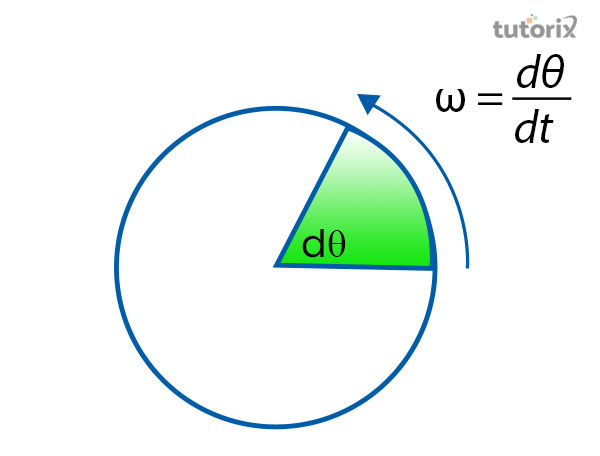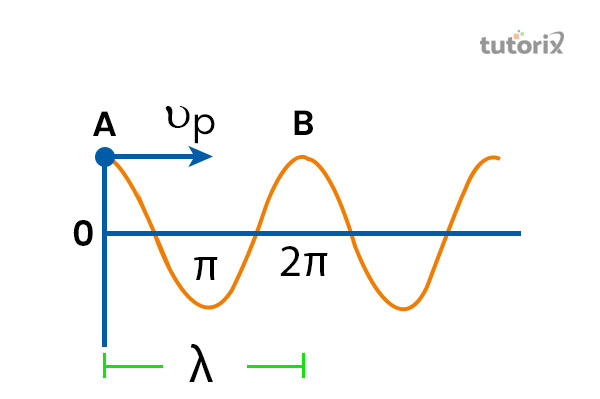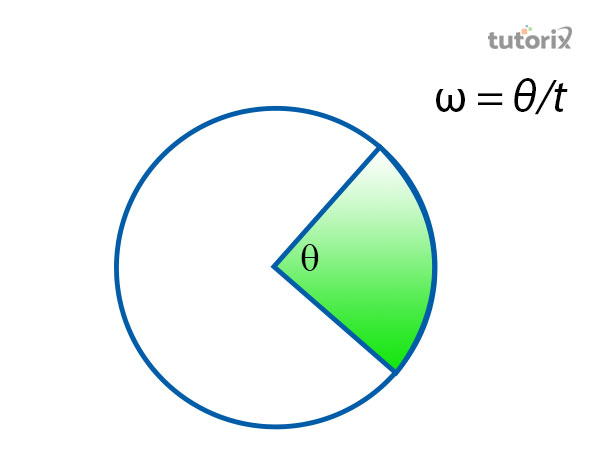# Period Angular Frequency

## Introduction

According to the terminology of physics, angular frequency is defined as the measurement of the angular displacement on the basis per unit of the time. In order to request this concept in a better-described version, proper definitions along with examples of the angular frequency have been included. Being focused on the conciliation of the wave, the formula of the angular frequency has been developed that includes the rate of change of the angular displacement.

## What is Angular Frequency?

An object or a body when sets free to rotate about a given axis, the object starts to make angular oscillation. This oscillation built up the velocity of an angular displacement of the object that can be defined as based on the movement of a segment similar to the change in angular position of the object.Figure 1: Angular displacement that creates an angular frequency

• Based on this concept, angular frequencies are generally known by the term circular or redial frequency including the measurement of the angular displacement based on each unit of time.
• The frequency of a periodic process that is expressed in radius per second is considered to be equal to the sinusoidal waveform that is charged based on each unit of time (Abouelregal & Marin, 2020).
• The frequency of this rotational object is generally measured on a basis of the number of the reputation of an event per unit of time.

## Angular Frequency of a Wave

The frequency of a rotational object is defined as a particular state of the object that is represented by a number of the complete cycles of passing a point by a wave in unit time. In order to determine the number of times, one needs to determine whether the wave passes a singular point.

The measurement of the angular frequency of a wave, therefore, includes the time period within which a complete cycle of a wave to pass a point is referred to. Based on this particular concept, the angular frequency has been known by the term angular displacement of any particle situated in the motion of the wave that crosses the stable point as per each unit of time.Figure 2: Angular frequency of a wave

On consideration of the above figure, it can be seen that the displacement of one element from a harmonic wave along with a particular string moves towards a particular direction that is fixed with respect to the time.

In order to determine the angular frequency of a wave, one must keep in mind that the string element is subjected to move up and down by maintaining a simple harmonic motion.

## Relation of the Angular Frequency with Time

Denoted by the particular letter T, it signifies the amount of time that is taken by the rotating object to complete a singular rotation based on the axis. In the case of vibration that is represented as wave motion, the time period is considered the amount of time the vibration passes through the stable point (Wardani, 2020).

These attributes such as time period and the angular frequency share a mathematical relationship that is expressed by the equation of.

T = 2m/qB

In this equation, the m represents the mass of the electron and q is the charge whereas the B is the representation of "the magnetic field in the religion". On the other hand, the time period of a rotational object is represented by the equation that is

T = 1/f

In this equation, the frequency is represented by the letter f and the T is the representative of the Time period.

## The Formula of Angular Frequency

The frequency of rotation refers to the count of the rotational moves that have been taken by the object within a certain amount of time. To represent the calculation of the angular frequency, the formula that is developed is f = 1/T (Wardani, 2020). For finding the angular rotation of the earth, the considered time period is 365 days. Determining this fact, the formula for calculating the angular frequency of the earth is

f =1/365

In some cases, the angular frequency formula is represented as "ω = 2πf", where the angular frequency is associated with the oscillation frequency of the moving object that is multiplied by the specific angle based on which the object generally forms the orbit of rotationFigure 3: Angular frequency

Based on this particular concept a general formula has been formed that is

ω = θ/t

This specific concept is represented in the above figure. On the context of the above figure, θ is considered as the angle that is determined by the rotating object and t represents the time that is taken by the moving object for travelling through the particular angle that is θ.

## Examples of Angular Frequency

In order to understand the formula through an example, it has been imagined that a person is sitting on top of a Ferris wheel. It has been observed by the person that the wheel completes one-quarter of its rotation in 15 seconds. In such cases, one has two approaches to finding out the angular frequency of the Ferris wheel.

According to the first formula, if the condition is considered if ¼ rotation is taking 15 seconds, the full rotation of the wheel will be completed by the time (4*15=) 60 seconds.

Based on this, the frequency of rotation f will be equivalent to 1/60s-1, thus the angular frequency will be ω = 2πf, equivalent to π/30.

Secondly, the person sits atop the wheel moves through π/2 radians in 15s, which leads to the formula of ω = (π/2)/15, equivalent to π/30.

## Conclusion

A scalar quantity of a rotational object is generally defined by the term angular frequency. It has been observed that the formula of the angular frequency is dependable on the attributes like the time period that is referred to as the particular axis based on which the object gets the velocity and the angular displacement. Based on the calculation of how frequently the object rockets or passes the fixed axis per unit of time helps to develop the formula that is ω=2πf

## FAQs

Q1. What is the difference between frequency and angular frequency?

The number of circles completed by the rotating object at a time defines frequency, the angular displacement observed in the circulatory motion of the object is referred to as the angular frequency.

Q2. What does the angular frequency of a spring depend on?

The angular frequency of spring is generally based on the consent force and the mass of the electron of the object and not on the amplitude.

Q3. Does the angular frequency share dependency with the spring constant?

It is determined in physics that the angular frequency of an object is constant thus, the angular frequency of a spring depends on the mass of the object and the spring constant.

Q4. What is the difference between angular velocity and frequency?

Angular velocity is equivalent to the product of frequency and the constant 2π whereas angular frequency is defined by the speed of the rotation per unit of the time.

## References

### Journals

Abouelregal, A. E., & Marin, M. (2020). The size-dependent thermoelastic vibrations of nanobeams are subjected to harmonic excitation and rectified sine wave heating. Mathematics, 8(7), 1128. Retrieved from: https://www.mdpi.com

Wardani, F. (2020, April). An analysis of student's concepts understanding about simple harmonic motion: Study in vocational high school. In Journal of Physics: Conference Series (Vol. 1511, No. 1, p. 012079). IOP Publishing. Retrieved from: http://repo.unida.gontor.ac

Websites

En.universaldenker.org, (2022). Phase Velocity of a Wave. Retrieved from: https://en.universaldenker.org [Retrieved on: 7th June 2022]

Lambdageeks.com, (2022). Angular Frequency Simple Harmonic Motion: Exhaustive Insights. Retrieved from: https://lambdageeks.com [Retrieved on: 7th June 2022]